# simulate

Monte Carlo simulation of conditional variance models

## Syntax

``V = simulate(Mdl,numObs)``
``V = simulate(Mdl,numObs,Name,Value)``
``````[V,Y] = simulate(___)``````

## Description

example

````V = simulate(Mdl,numObs)` simulates a `numObs`-period conditional variance path from the fully specified conditional variance model `Mdl`. `Mdl` can be a `garch`, `egarch`, or `gjr` model.```

example

````V = simulate(Mdl,numObs,Name,Value)` simulates conditional variance paths with additional options specified by one or more `Name,Value` pair arguments. For example, you can generate multiple sample paths or specify presample innovation paths.```

example

``````[V,Y] = simulate(___)``` additionally simulates response paths using any of the input arguments in the previous syntaxes.```

## Examples

collapse all

Simulate conditional variance and response paths from a GARCH(1,1) model.

Specify a GARCH(1,1) model with known parameters.

`Mdl = garch('Constant',0.01,'GARCH',0.7,'ARCH',0.2);`

Simulate 500 sample paths, each with 100 observations.

```rng default; % For reproducibility [V,Y] = simulate(Mdl,100,'NumPaths',500); figure subplot(2,1,1) plot(V) title('Simulated Conditional Variances') subplot(2,1,2) plot(Y) title('Simulated Responses')```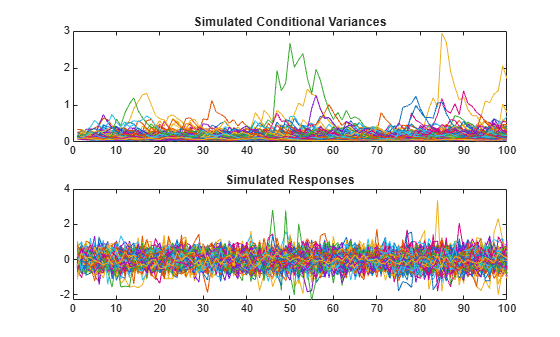The simulated responses look like draws from a stationary stochastic process.

Plot the 2.5th, 50th (median), and 97.5th percentiles of the simulated conditional variances.

```lower = prctile(V,2.5,2); middle = median(V,2); upper = prctile(V,97.5,2); figure plot(1:100,lower,'r:',1:100,middle,'k',... 1:100,upper,'r:','LineWidth',2) legend('95% Interval','Median') title('Approximate 95% Intervals')```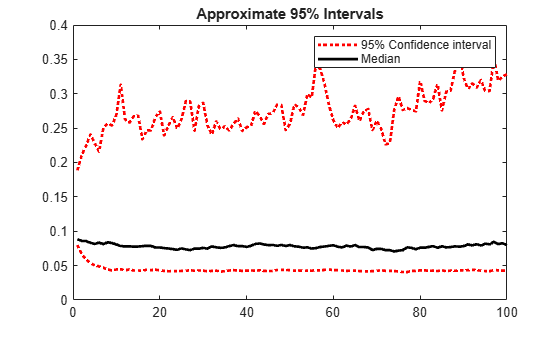The intervals are asymmetric due to positivity constraints on the conditional variance.

Simulate conditional variance and response paths from an EGARCH(1,1) model.

Specify an EGARCH(1,1) model with known parameters.

```Mdl = egarch('Constant',0.001,'GARCH',0.7,'ARCH',0.2,... 'Leverage',-0.3);```

Simulate 500 sample paths, each with 100 observations.

```rng default; % For reproducibility [V,Y] = simulate(Mdl,100,'NumPaths',500); figure subplot(2,1,1) plot(V) title('Simulated Conditional Variances') subplot(2,1,2) plot(Y) title('Simulated Responses (Innovations)')```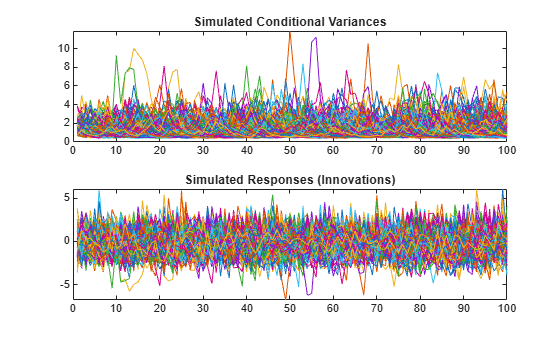The simulated responses look like draws from a stationary stochastic process.

Plot the 2.5th, 50th (median), and 97.5th percentiles of the simulated conditional variances.

```lower = prctile(V,2.5,2); middle = median(V,2); upper = prctile(V,97.5,2); figure plot(1:100,lower,'r:',1:100,middle,'k',... 1:100, upper,'r:','LineWidth',2) legend('95% Interval','Median') title('Approximate 95% Intervals')```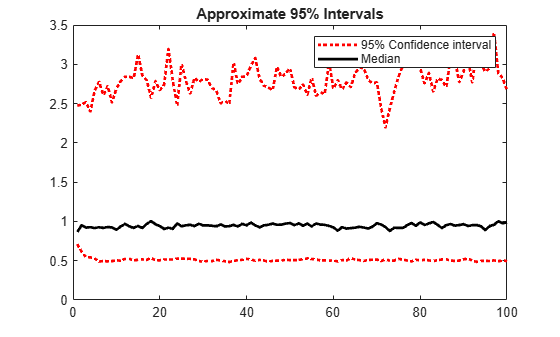The intervals are asymmetric due to positivity constraints on the conditional variance.

Simulate conditional variance and response paths from a GJR(1,1) model.

Specify a GJR(1,1) model with known parameters.

```Mdl = gjr('Constant',0.001,'GARCH',0.7,'ARCH',0.2,... 'Leverage',0.1);```

Simulate 500 sample paths, each with 100 observations.

```rng default; % For reproducibility [V,Y] = simulate(Mdl,100,'NumPaths',500); figure subplot(2,1,1) plot(V) title('Simulated Conditional Variances') subplot(2,1,2) plot(Y) title('Simulated Responses (Innovations)')```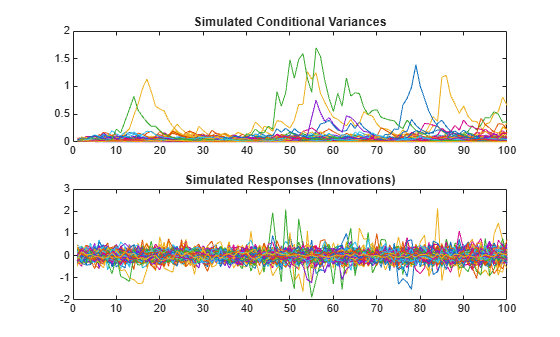The simulated responses look like draws from a stationary stochastic process.

Plot the 2.5th, 50th (median), and 97.5th percentiles of the simulated conditional variances.

```lower = prctile(V,2.5,2); middle = median(V,2); upper = prctile(V,97.5,2); figure plot(1:100,lower,'r:',1:100,middle,'k',... 1:100, upper,'r:','LineWidth',2) legend('95% Interval','Median') title('Approximate 95% Intervals')```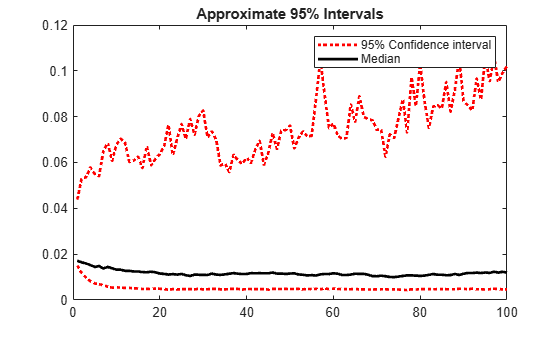The intervals are asymmetric due to positivity constraints on the conditional variance.

Simulate conditional variances of the daily NASDAQ Composite Index returns for 500 days. Use the simulations to make forecasts and approximate 95% forecast intervals. Compare the forecasts among GARCH(1,1), EGARCH(1,1), and GJR(1,1) fits.

Load the NASDAQ data included with the toolbox. Convert the index to returns.

```load Data_EquityIdx nasdaq = DataTable.NASDAQ; r = price2ret(nasdaq); T = length(r);```

Fit GARCH(1,1), EGARCH(1,1), and GJR(1,1) models to the entire data set. Infer conditional variances to use as presample conditional variances for the forecast simulation.

```Mdl = cell(3,1); % Preallocation Mdl{1} = garch(1,1); Mdl{2} = egarch(1,1); Mdl{3} = gjr(1,1); EstMdl = cellfun(@(x)estimate(x,r,'Display','off'),Mdl,... 'UniformOutput',false); v0 = cellfun(@(x)infer(x,r),EstMdl,'UniformOutput',false);```

`EstMdl` is 3-by-1 cell vector. Each cell is a different type of estimated conditional variance model, e.g., `EstMdl{1}` is an estimated GARCH(1,1) model. `V0` is a 3-by-1 cell vector, and each cell contains the inferred conditional variances from the corresponding, estimated model.

Simulate 1000 samples paths with 500 observations each. Use the observed returns and inferred conditional variances as presample data.

```vSim = cell(3,1); % Preallocation for j = 1:3 rng default; % For reproducibility vSim{j} = simulate(EstMdl{j},500,'NumPaths',1000,'E0',r,'V0',v0{j}); end```

`vSim` is a 3-by-1 cell vector, and each cell contains a 500-by-1000 matrix of simulated conditional variances generated from the corresponding, estimated model.

Plot the simulation mean forecasts and approximate 95% forecast intervals, along with the conditional variances inferred from the data.

```lower = cellfun(@(x)prctile(x,2.5,2),vSim,'UniformOutput',false); upper = cellfun(@(x)prctile(x,97.5,2),vSim,'UniformOutput',false); mn = cellfun(@(x)mean(x,2),vSim,'UniformOutput',false); datesPlot = dates(end - 250:end); datesFH = dates(end) + (1:500)'; h = zeros(3,4); figure for j = 1:3 col = zeros(1,3); col(j) = 1; h(j,1) = plot(datesPlot,v0{j}(end-250:end),'Color',col); hold on h(j,2) = plot(datesFH,mn{j},'Color',col,'LineWidth',3); h(j,3:4) = plot([datesFH datesFH],[lower{j} upper{j}],':',... 'Color',col,'LineWidth',2); end hGCA = gca; plot(datesFH(1)*[1 1],hGCA.YLim,'k--'); datetick; axis tight; h = h(:,1:3); legend(h(:),'GARCH - Inferred','EGARCH - Inferred','GJR - Inferred',... 'GARCH - Sim. Mean','EGARCH - Sim. Mean','GJR - Sim. Mean',... 'GARCH - 95% Fore. Int.','EGARCH - 95% Fore. Int.',... 'GJR - 95% Fore. Int.','Location','NorthEast') title('Simulated Conditional Variance Forecasts') hold off```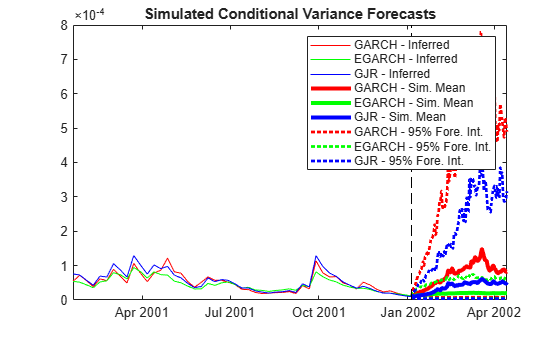## Input Arguments

collapse all

Conditional variance model without any unknown parameters, specified as a `garch`, `egarch`, or `gjr` model object.

`Mdl` cannot contain any properties that have `NaN` value.

Sample path length, specified as a positive integer. That is, the number of random observations to generate per output path. `V` and `Y` have `numObs` rows.

### Name-Value Arguments

Specify optional pairs of arguments as `Name1=Value1,...,NameN=ValueN`, where `Name` is the argument name and `Value` is the corresponding value. Name-value arguments must appear after other arguments, but the order of the pairs does not matter.

Before R2021a, use commas to separate each name and value, and enclose `Name` in quotes.

Example: `'numPaths',1000,'E0',[0.5; 0.5]` specifies to generate `1000` sample paths and to use `[0.5; 0.5]` as presample innovations per path.

Number of sample paths to generate, specified as the comma-separated pair consisting of `'NumPaths'` and a positive integer. `V` and `Y` have `NumPaths` columns.

Example: `'NumPaths',1000`

Data Types: `double`

Presample innovations, specified as the comma-separated pair consisting of `'E0'` and a numeric column vector or matrix. The presample innovations provide initial values for the innovations process of the conditional variance model `Mdl`. The presample innovations derive from a distribution with mean 0.

`E0` must contain at least `Mdl.Q` elements or rows. If `E0` contains extra rows, `simulate` uses the latest `Mdl.Q` only.

The last element or row contains the latest presample innovation.

• If `E0` is a column vector, it represents a single path of the underlying innovation series. `simulate` applies `E0` to each simulated path.

• If `E0` is a matrix, then each column represents a presample path of the underlying innovation series. `E0` must have at least `NumPaths` columns. If `E0` has more columns than necessary, `simulate` uses the first `NumPaths` columns only.

The defaults are:

• For GARCH(P,Q) and GJR(P,Q) models, `simulate` sets any necessary presample innovations to an independent sequence of disturbances with mean zero and standard deviation equal to the unconditional standard deviation of the conditional variance process.

• For EGARCH(P,Q) models, `simulate` sets any necessary presample innovations to an independent sequence of disturbances with mean zero and variance equal to the exponentiated unconditional mean of the logarithm of the EGARCH variance process.

Example: `'E0',[0.5; 0.5]`

Positive presample conditional variance paths, specified as a numeric vector or matrix. `V0` provides initial values for the conditional variances in the model.

• If `V0` is a column vector, then `simulate` applies it to each output path.

• If `V0` is a matrix, then it must have at least `NumPaths` columns. If `V0` has more columns than necessary, `simulate` uses the first `NumPaths` columns only.

• For GARCH(P,Q) and GJR(P,Q) models:

• `V0` must have at least `Mdl.P` rows to initialize the variance equation.

• By default, `simulate` sets any necessary presample variances to the unconditional variance of the conditional variance process.

• For EGARCH(P,Q) models, `simulate`:

• `V0` must have at least `max(Mdl.P,Mdl.Q)` rows to initialize the variance equation.

• By default, `simulate` sets any necessary presample variances to the exponentiated unconditional mean of the logarithm of the EGARCH variance process.

If the number of rows in `V0` exceeds the number necessary, then `simulate` uses the latest, required number of observations only. The last element or row contains the latest observation.

Example: `'V0',[1; 0.5]`

Data Types: `double`

Notes

• If `E0` and `V0` are column vectors, `simulate` applies them to every column of the outputs `V` and `Y`. This application allows simulated paths to share a common starting point for Monte Carlo simulation of forecasts and forecast error distributions.

• `NaN`s indicate missing values. `simulate` removes missing values. The software merges the presample data (`E0` and `V0`), and then uses list-wise deletion to remove any rows containing at least one `NaN`. Removing `NaN`s in the data reduces the sample size. Removing `NaNs` can also create irregular time series.

• `simulate` assumes that you synchronize presample data such that the latest observation of each presample series occurs simultaneously.

## Output Arguments

collapse all

Simulated conditional variance paths of the mean-zero innovations associated with `Y`, returned as a numeric column vector or matrix.

`V` is a `numObs`-by-`NumPaths` matrix, in which each column corresponds to a simulated conditional variance path. Rows of `V` are periods corresponding to the periodicity of `Mdl`.

Simulated response paths, returned as a numeric column vector or matrix. `Y` usually represents a mean-zero, heteroscedastic time series of innovations with conditional variances given in `V` (a continuation of the presample innovation series `E0`).

`Y` can also represent a time series of mean-zero, heteroscedastic innovations plus an offset. If `Mdl` includes an offset, then `simulate` adds the offset to the underlying mean-zero, heteroscedastic innovations so that `Y` represents a time series of offset-adjusted innovations.

`Y` is a `numObs`-by-`NumPaths` matrix, in which each column corresponds to a simulated response path. Rows of `Y` are periods corresponding to the periodicity of `Mdl`.

 Bollerslev, T. “Generalized Autoregressive Conditional Heteroskedasticity.” Journal of Econometrics. Vol. 31, 1986, pp. 307–327.

 Bollerslev, T. “A Conditionally Heteroskedastic Time Series Model for Speculative Prices and Rates of Return.” The Review of Economics and Statistics. Vol. 69, 1987, pp. 542–547.

 Box, G. E. P., G. M. Jenkins, and G. C. Reinsel. Time Series Analysis: Forecasting and Control. 3rd ed. Englewood Cliffs, NJ: Prentice Hall, 1994.

 Enders, W. Applied Econometric Time Series. Hoboken, NJ: John Wiley & Sons, 1995.

 Engle, R. F. “Autoregressive Conditional Heteroskedasticity with Estimates of the Variance of United Kingdom Inflation.” Econometrica. Vol. 50, 1982, pp. 987–1007.

 Glosten, L. R., R. Jagannathan, and D. E. Runkle. “On the Relation between the Expected Value and the Volatility of the Nominal Excess Return on Stocks.” The Journal of Finance. Vol. 48, No. 5, 1993, pp. 1779–1801.

 Hamilton, J. D. Time Series Analysis. Princeton, NJ: Princeton University Press, 1994.

 Nelson, D. B. “Conditional Heteroskedasticity in Asset Returns: A New Approach.” Econometrica. Vol. 59, 1991, pp. 347–370.